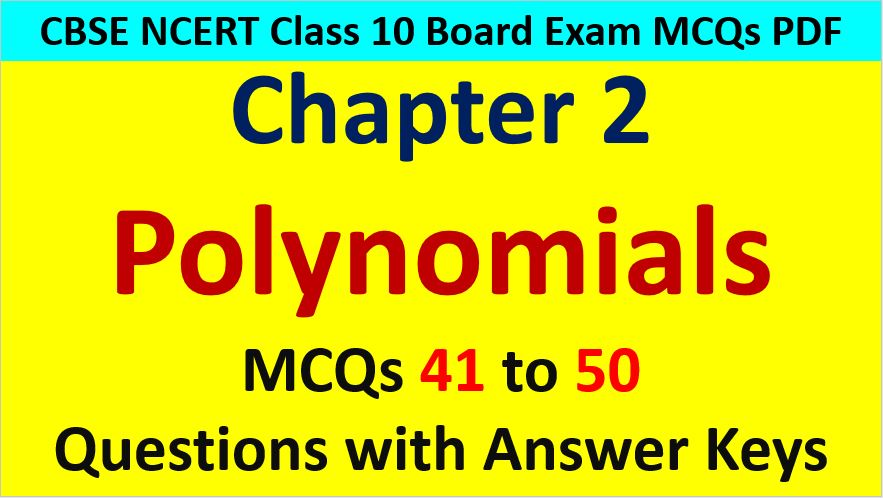Friday, October 22, 2021
Home > CBSE Class 10 > CBSE Class 10 Math Polynomials MCQ Questions with Answer Keys

# CBSE Class 10 Math Polynomials MCQ Questions with Answer KeysHi students, Welcome to AMBiPi (Amans Maths Blogs). In this article, you will get CBSE Class 10 Math Polynomials MCQ Questions with Answer Keys. You can download this PDF and save it in your mobile device or laptop etc.

Polynomials CBSE Class 10 MCQ Question No 41:

If one root of the polynomial is f(x) = 5x2 + 13x + k is reciprocal of other, then the value of k is

Option A : 0

Option B : 5

Option C : 1/6

Option D : 6

Option B : 5

Polynomials CBSE Class 10 MCQ Question No 42:

If the product of two zeroes of the polynomial f(x) = 2x3 + 6x2 – 4x + 9 is 3, then its third zero is

Option A : 3/2

Option B : -3/2

Option C : 9/2

Option D : -9/2

Option B : -9/2

Polynomials CBSE Class 10 MCQ Question No 43:

If f(x) = ax2 + bx + c has no real roots and a + b + c < 0, then

Option A : c = 0

Option B : c > 0

Option C : c < 0

Option D : None of these

Option C : c < 0

Polynomials CBSE Class 10 MCQ Question No 44:

The number of real zeroes of the polynomial x4 + 4x2 + 5 is

Option A : One real zero

Option B : Two real zero

Option C : Three real zero

Option D : No real zero

Option D : No real zero

Polynomials CBSE Class 10 MCQ Question No 45:

If the polynomials 2x3 + ax2 + 3x – 5 and x3 + x2 – 4x + a leave the same remainder when divided by (x – 2), then the value of a is

Option A : 3/13

Option B : 3/17

Option C : -13/3

Option D : -3/13

Option C : -13/3

Polynomials CBSE Class 10 MCQ Question No 46:

When a polynomial 2x3 + ax2 + 3x – 5 is divided by a polynomial (x + 1), then the remainder will be

Option A : -1

Option B : 1

Option C : 2

Option D : None of these

Option A : -1

Polynomials CBSE Class 10 MCQ Question No 47:

If x + 1/x = 3, then x2 + 1/x2 is equal to

Option A : 9

Option B : 82/9

Option C : 7

Option D : 11

Option C : 7

Polynomials CBSE Class 10 MCQ Question No 48:

If (x – 2) is a factor of (x2 + 3qx – 2q), then the value of q is

Option A : 2

Option B : -2

Option C : 1

Option D : -1

Option D : -1

Polynomials CBSE Class 10 MCQ Question No 49:

If a + b = 10 and ab = 21, then the value of a3 + b3 is

Option A : 370

Option B : 365

Option C : 360

Option D : 355

Option A : 370

Polynomials CBSE Class 10 MCQ Question No 50:

Factors of x3 + 3x2 + 3x – 7 are

Option A : (x – 1)(x2 + 4x + 7)

Option B : (x + 1)(x2 + 4x + 7)

Option C : (x + 1)(x2 + 4x – 7)

Option D : (x – 1)(x2 – 4x + 7)